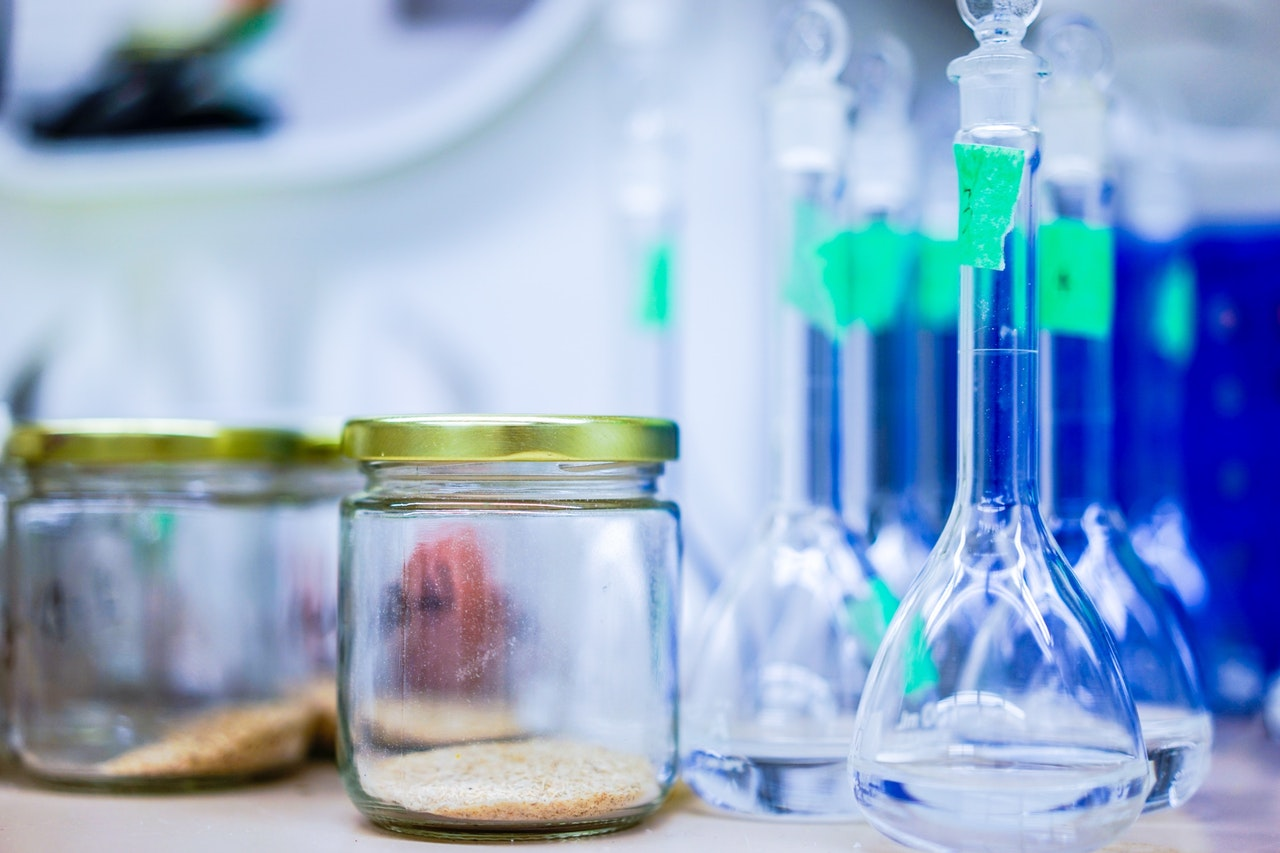Post-Lab Report: Mole | My Paper Hub

# Post-Lab Report: Mole

Stoichiometry of a Chemical ReactionPost-Lab Report(a) Report the collected data with the correct uncertainty. Trial Mass H2C2O4 (g) Initial Volume NaOH(mL) Final Volume NaOH(mL) (Na...Read More

~Posted on Jun 2018

Type Of Paper

Pages

- + 275 words## Stoichiometry of a Chemical ReactionPost-Lab Repo...

Stoichiometry of a Chemical Reaction

Post-Lab Report

(a) Report the collected data with the correct uncertainty.

 Trial Mass H2C2O4 (g) Initial Volume NaOH(mL) Final Volume NaOH(mL) (NaOH) mol/L 1 0.1 3 20.4 0.100 2 0.1996 3.5 34.6 0.100 3 0.1959 2.5 33.5 0.100

(b) Calculate the moles of each reactant used (Show one sample calculation).

Mole = Mass/Molar massMolar Mass of (H2C2O4)          (H=1*2 + C=2*12 + O=4*16) =90 g/mol

Mole = 0.1g/90 g/mol = 0.00111

 Trial Mol H2C2O4 Mol NaOH Mol (H2C2O4)/ mol (NaOH) 1 0.00111 0.03 0.037 2 0.00223 0.035 0.064 3 0.00227 0.025 0.091

(c) Report the balanced chemical equation.H2C2O4 (aq) +NaOH(aq)                         Na(g) + H3C2O5 (g)

Report

The balanced chemical equation is an indication that the both reduction and addition took place. The sodium hydroxide was reduced into the sodium metal, thus reducing its mass. However, the crystalline carboxyl acid received two elements of carbon and four elements of oxygen resulting into a different compound H3C2O5 (g) which is gaseous in nature thus increasing in weight.

## Dedicated to Results## Raise that GPA.